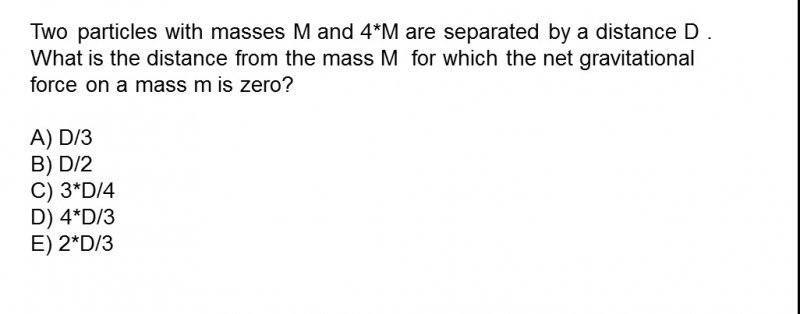• Maged Saeed

## Homework Equations

$$F=\frac{GMm}{d^2}$$

## The Attempt at a Solution

I'm stuck with this problem , because I cannot imagine it properly.
If I say that the mass m is in between the two masses , I cannot find d in the choices ,, Any help please!The prompt gives you that the two large masses are separated by D, and that the small mass is somewhere between them, meaning that you are being asked for "d" in terms of "D."

•Maged Saeed
The mass in between would have a net gravitational force equal to :
$$\frac{GMm}{d^2}-\frac{G(4M)m}{(D-d)^2}$$
and that should be ZERO.
Then solving for d won't lead to one of the choices!

What equation are you solving?

•Maged Saeed
The equation is of the net gravitational force acting on smaller mass which should be zero.

WRITE the equation.

•Maged Saeed
$$\frac{GMm}{d^2}-\frac{G(4M)m}{(D-d)^2}=0$$

Can you eliminate GMm? Yes. Do so.

•Maged Saeed
Oh,,, I got it ...
Thanks

Take things one step at a time, and they'll usually fall into place for you.

•Maged Saeed